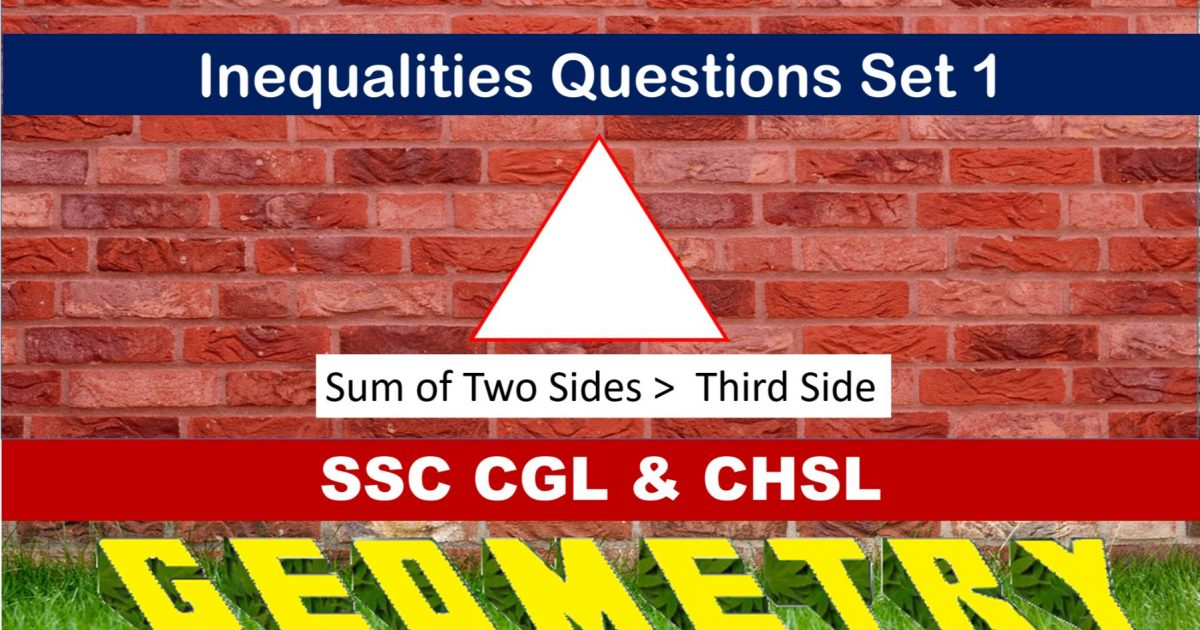Monday, September 27, 2021
Home > geometry > SSC CGL Geometry Inequality Set 1

# SSC CGL Geometry Inequality Set 1SSC CGL Geometry Inequality Set 1 : Ques No 1

In an isosceles triangle FGH, FG < 3 cm and GH = 8 cm. Then the correct relation is

Options:

A. GH = FH

B. GH < FH

C. GF = GH

D. FH > GH

Solution:

SSC CGL Geometry Inequality Set 1 : Ques No 2

The sum of three altitudes of a triangle is

Options:

A. equal to the sum of three sides

B. twice the sum of sides

C. greater than the sum of the sides

D. less than the sum of the sides

Solution:

SSC CGL Geometry Inequality Set 1 : Ques No 3

How many triangles can be formed by taking any three from the four line segments of lengths 2 cm, 3 cm, 5 cm and 6 cm?

Options:

A. 1

B. 2

C. 3

D. 4

Solution:

SSC CGL Geometry Inequality Set 1 : Ques No 4

If A, B and C are three points lying on the same place and AB = 5 cm and BC = 10 cm, then the possible length of AC (in cm) is

Options:

A. 15

B. 5

C. 3

D. 6

Solution:

SSC CGL Geometry Inequality Set 1 : Ques No 5

In the triangle ABC, AD, BE and CF are three altitudes and AD and BE intersect at G with BE + EG = BG. Then,

Options:

A. FC + CG = FG

B. CF + FG = CG

C. CG + GF = CF

D. CF – FG = CG

Solution:

SSC CGL Geometry Inequality Set 1 : Ques No 6

Determine which three lengths can be the measure of the sides of a triangle.

Options:

A. 14cm, 3cm, 18cm

B. 9cm, 7cm, 15cm

C. 2cm, 14cm, 18cm

D. 6cm, 9cm, 15cm

SSC CGL Geometry Inequality Set 1 : Ques No 7

Determine which three lengths can not be the measure of the sides of a triangle

Options:

A. 2m, 5m, 6m

B. 17m, 8m, 10m

C. 22m, 20m, 5m

D. 15m, 4m, 20m

SSC CGL Geometry Inequality Set 1 : Ques No 8

The measure of two sides of a triangle are 8 and 15, find the range of the possible values for the third side

Options:

A. 9 < x < 16

B. 7 < x < 16

C. 7 < x < 23

D. 9 < x < 23

SSC CGL Geometry Inequality Set 1 : Ques No 9

Look at the triangle. What is NOT a possible value of x?Options:

A. 6

B. 4

C. 7 < x < 23

D. 1

SSC CGL Geometry Inequality Set 1 : Ques No 10

Which numbers could represent the lengths of the sides of a triangle?

Options:

A. 5, 9, 14

B. 7, 7, 15

C. 1, 2, 4

D. 3, 6, 8

SSC CGL Geometry Inequality Set 1 : Ques No 11

Which set of numbers represents the lengths of the sides of a triangle?

Options:

A. 5, 8, 13

B. 6, 17, 22

C. 16, 24, 7

D. 26, 8, 15

SSC CGL Geometry Inequality Set 1 : Ques No 12

Elina is cutting a triangular piece of tile. If the triangle is scalene, which set of numbers could represent the lengths of the sides?

Options:

A. 2, 4, 7

B. 4, 5, 6

C. 3, 5, 8

D. 5, 5, 8

SSC CGL Geometry Inequality Set 1 : Ques No 13

Which set can not represent the lengths of the sides of a triangle?

Options:

A. 4, 5, 6

B. 5, 5, 11

C. 7, 7, 12

D. 8, 8, 8

SSC CGL Geometry Inequality Set 1 : Ques No 14

Which set could not represent the lengths of the sides of a triangle?

Options:

A. 3, 4, 5,

B. 2, 5, 9

C. 5, 10, 12

D. 7, 9, 11

SSC CGL Geometry Inequality Set 1 : Ques No 15

In ABC, AB = 5 feet and BC = 3 feet. Which inequality represents all possible values for the
length of AC, in feet?

Options:

A. 2 <= AC <= 8

B. 2 < AC < 8

C. 3 <= AC <= 7

D. 3 < AC < 7